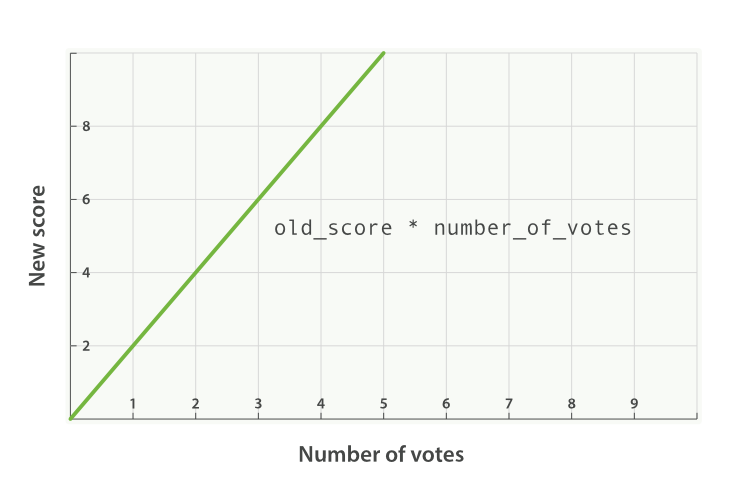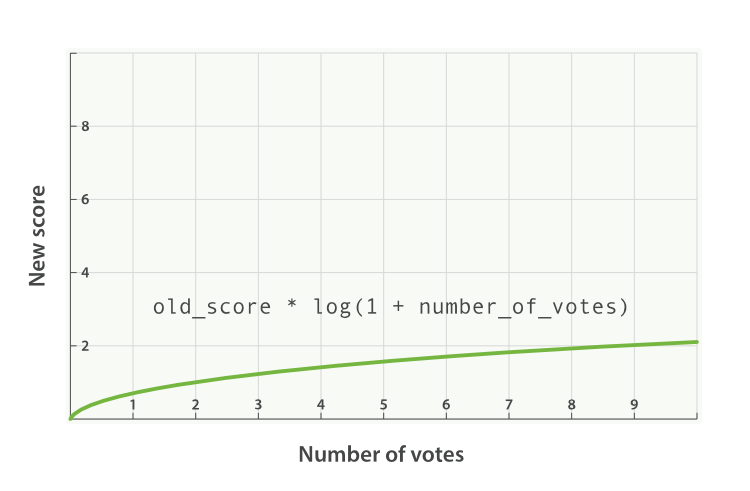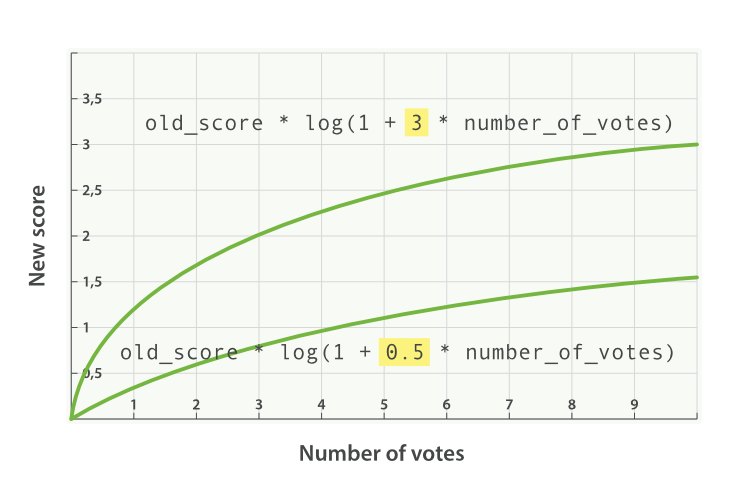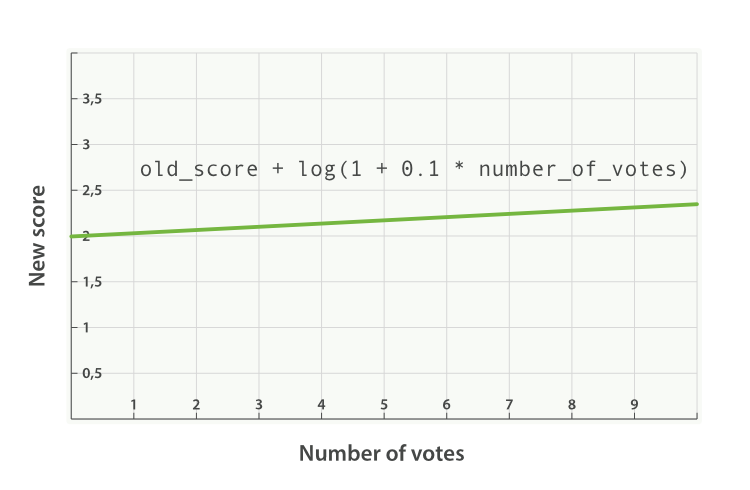## Boosting by Popularityedit

Imagine that we have a website that hosts blog posts and enables users to vote for the blog posts that they like. We would like more-popular posts to appear higher in the results list, but still have the full-text score as the main relevance driver. We can do this easily by storing the number of votes with each blog post:

```PUT /blogposts/post/1
{
"content": "In this post we will talk about...",
}```

At search time, we can use the `function_score` query with the `field_value_factor` function to combine the number of votes with the full-text relevance score:

```GET /blogposts/post/_search
{
"query": {
"function_score": {
"query": {
"multi_match": {
"query":    "popularity",
"fields": [ "title", "content" ]
}
},
"field_value_factor": {
}
}
}
}```
 The `function_score` query wraps the main query and the function we would like to apply. The main query is executed first. The `field_value_factor` function is applied to every document matching the main `query`. Every document must have a number in the `votes` field for the `function_score` to work.

In the preceding example, the final `_score` for each document has been altered as follows:

`new_score = old_score * number_of_votes`

This will not give us great results. The full-text `_score` range usually falls somewhere between 0 and 10. As can be seen in Figure 29, “Linear popularity based on an original `_score` of `2.0`, a blog post with 10 votes will completely swamp the effect of the full-text score, and a blog post with 0 votes will reset the score to zero.Figure 29. Linear popularity based on an original `_score` of `2.0`

### modifieredit

A better way to incorporate popularity is to smooth out the `votes` value with some `modifier`. In other words, we want the first few votes to count a lot, but for each subsequent vote to count less. The difference between 0 votes and 1 vote should be much bigger than the difference between 10 votes and 11 votes.

A typical `modifier` for this use case is `log1p`, which changes the formula to the following:

`new_score = old_score * log(1 + number_of_votes)`

The `log` function smooths out the effect of the `votes` field to provide a curve like the one in Figure 30, “Logarithmic popularity based on an original `_score` of `2.0`.Figure 30. Logarithmic popularity based on an original `_score` of `2.0`

The request with the `modifier` parameter looks like the following:

```GET /blogposts/post/_search
{
"query": {
"function_score": {
"query": {
"multi_match": {
"query":    "popularity",
"fields": [ "title", "content" ]
}
},
"field_value_factor": {
"modifier": "log1p"
}
}
}
}```
 Set the `modifier` to `log1p`.

The available modifiers are `none` (the default), `log`, `log1p`, `log2p`, `ln`, `ln1p`, `ln2p`, `square`, `sqrt`, and `reciprocal`. You can read more about them in the `field_value_factor` documentation.

### factoredit

The strength of the popularity effect can be increased or decreased by multiplying the value in the `votes` field by some number, called the `factor`:

```GET /blogposts/post/_search
{
"query": {
"function_score": {
"query": {
"multi_match": {
"query":    "popularity",
"fields": [ "title", "content" ]
}
},
"field_value_factor": {
"modifier": "log1p",
"factor":   2
}
}
}
}```
 Doubles the popularity effect

Adding in a `factor` changes the formula to this:

`new_score = old_score * log(1 + factor * number_of_votes)`

A `factor` greater than `1` increases the effect, and a `factor` less than `1` decreases the effect, as shown in Figure 31, “Logarithmic popularity with different factors”.Figure 31. Logarithmic popularity with different factors

### boost_modeedit

Perhaps multiplying the full-text score by the result of the `field_value_factor` function still has too large an effect. We can control how the result of a function is combined with the `_score` from the query by using the `boost_mode` parameter, which accepts the following values:

`multiply`
Multiply the `_score` with the function result (default)
`sum`
Add the function result to the `_score`
`min`
The lower of the `_score` and the function result
`max`
The higher of the `_score` and the function result
`replace`
Replace the `_score` with the function result

If, instead of multiplying, we add the function result to the `_score`, we can achieve a much smaller effect, especially if we use a low `factor`:

```GET /blogposts/post/_search
{
"query": {
"function_score": {
"query": {
"multi_match": {
"query":    "popularity",
"fields": [ "title", "content" ]
}
},
"field_value_factor": {
"modifier": "log1p",
"factor":   0.1
},
"boost_mode": "sum"
}
}
}```
 Add the function result to the `_score`.

The formula for the preceding request now looks like this (see Figure 32, “Combining popularity with `sum`):

`new_score = old_score + log(1 + 0.1 * number_of_votes)`Figure 32. Combining popularity with `sum`

### max_boostedit

Finally, we can cap the maximum effect that the function can have by using the `max_boost` parameter:

```GET /blogposts/post/_search
{
"query": {
"function_score": {
"query": {
"multi_match": {
"query":    "popularity",
"fields": [ "title", "content" ]
}
},
"field_value_factor": {
 Whatever the result of the `field_value_factor` function, it will never be greater than `1.5`.
The `max_boost` applies a limit to the result of the function only, not to the final `_score`.